Difference between revisions of "Mathematical programming"

The branch of mathematics concerned with the theory and methods for solving problems on finding the extrema of functions on sets defined by linear and non-linear constraints (equalities and inequalities) in a finite-dimensional vector space. Mathematical programming is a branch of operations research, which comprises a wide class of control problems the mathematical models of which are finite-dimensional extremum problems. The problems of mathematical programming find applications in various areas of human activity where it is necessary to choose one of the possible ways of action, e.g. in solving numerous problems of control and planning of production processes as well as in problems of design and long-term planning. The term "mathematical programming" is connected with the fact that the goal of solving various problems is choosing programs of action.

The mathematical formulation of the problem of mathematical programming is: To minimize a scalar function $\phi (x)$ of a vector argument on the set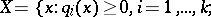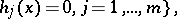where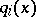and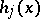are scalar functions. The function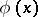is called the objective function, and also the quality criterion, the setis called the feasible set, or the set of plans, a solution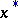of the mathematical programming problem is an optimum point (or vector), a point of global minimum and also an optimal plan.

In mathematical programming one customarily distinguishes the following branches. Linear programming: The objective function $\phi (x)$ and the constraintsandare linear. Quadratic programming: The objective function is quadratic and convex, while the feasible set is defined by linear equalities and inequalities. Convex programming: The objective function and the constraints are convex. Discrete programming: The solution is sought only at discrete, say integer, points of. Stochastic programming: In contrast to deterministic problems, here the data contain an element of indeterminacy. For example, in stochastic problems of minimization of a linear functionunder linear constraints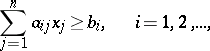the parameters, or only some of them, are random. The problems of linear, quadratic and convex programming have a common property: Local optimality implies global optimality. The so-called multi-extremum problems, for which the indicated property does not hold, are both considerably more difficult and less investigated.

At the basis of the theory of convex, and, in particular, linear and quadratic, programming lies the Kuhn–Tucker theorem, which gives necessary and sufficient conditions for the existence of an optimum point: In order thatbe an optimum point, i.e.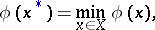it is necessary and sufficient that there exist a point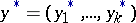such that the pair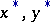be a saddle point of the Lagrange function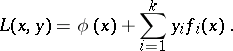The latter means that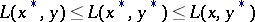for alland all. If the constraints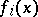are non-linear, the theorem is valid under some additional assumptions on the feasible set, for example, the existence of a pointsuch that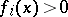,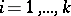(Slater's regularity condition).

Ifandare differentiable functions, then the following relations characterize a saddle point: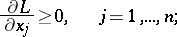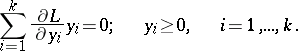Thus, the problem of convex programming reduces to the solution of a system of equations and inequalities.

On the basis of the Kuhn–Tucker theorem various iterative minimization methods were developed, which amount to searching for a saddle point of Lagrange's function.

In mathematical programming one of the main directions concerns computational methods for solving extremum problems. One of the widest used among these methods is the method of feasible directions. In this method a sequence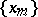of points of the setis constructed by the formula. At each iteration, to calculate the point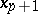one has to choose a direction (a vector)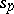and a step length (a number)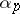. Forone selects from among the feasible directions (i.e. directions at the point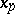, a small displacement along which does not lead out of the set) the one that makes an acute angle with the direction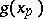of steepest descent of the objective function (with the vector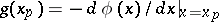ifis differentiable). Therefore, along the directionthe function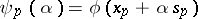is decreasing. The numberis determined from the conditions that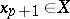and. To calculateatone defines the cone of feasible directions, given by a system of inequalities, and one formulates the problem (of linear programming) of finding the possible direction along which the objective function decreases most quickly. This problem is readily solved using, say, the standard simplex method. The step lengthis determined by solving the minimization problem for the function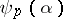. Under rather general assumptions it has been shown that the distance fromto the set of stationary points of the original problem (i.e. the set of points at which the conditions necessary for a local minimum ofonare satisfied) tends to zero as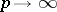. In the case when the original problem is a problem in convex programming, then, as,approaches the set of solutions (optimum points) of the original problem. The method of feasible directions admits an approximate solution of the indicated problems of determination ofand, and in this sense it is stable with respect to computational errors.

For feasible sets of special structure (from the point of view of the simplicity of choosing) one can apply minimization methods different from the method of feasible directions. Thus, in a gradient-projection method, as the descent direction at the pointone takes, where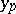is the projection of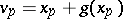,, on the set.

Ifis given by linear constraints, one has the rather promising method of the conditional gradient: One linearizes the objective function atby constructing the function, and then, minimizingon the set, one finds a minimum pointof it and one finally sets.

Minimization methods for non-smooth functions were successfully elaborated. One of the representatives of this class is the method of the generalized gradient. By definition, a generalized gradient atis any vector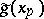such that the inequalityholds for all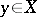. This method differs from the projection-gradient method in that for the point that is projected one takes.

Stochastic methods of minimization are also widely used. In the simplest variant of the method of random descent one takes fora random point on the-dimensional unit sphere with centre at the coordinate origin, and ifis a feasible direction and if, in addition,decreases along this direction, then a step is effected, i.e. one shifts to the point. In the opposite case, the procedure for selectingis carried out anew.

A characteristic feature of the computational aspect of the methods for solving the problems in mathematical programming is that the application of these methods is invariably connected with the utilization of electronic computers. The main reason for this is that the problems in mathematical programming that formalize situations of control of real systems involve a large amount of work which cannot be performed by manual computation.

One of the widespread methods for investigating problems in mathematical programming is the method of penalty functions (cf. Penalty functions, method of). This method essentially replaces the given mathematical programming problem by a sequence of parametric problems on unconditional minimization, such that when the parameter tends to infinity (in other cases, to zero) the solutions of these auxiliary problems converge to the solution of the original problem. Note that in an unconditional minimization problem every direction is feasible, and consequently the search forrequires less effort in comparison with the solution of the same problem by the method of feasible directions. (For example, in the method of steepest descent one takes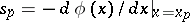.) The same is true concerning the search for.

An important direction of investigation in mathematical programming is the problem of stability. Here essential importance is attached to the study of the class of stable problems, that is, problems for which small perturbations (errors) in the data result in perturbations of the solutions that are also small. In the case of unstable problems an important role is reserved for a procedure of approximating the unstable problem by a sequence of stable problems — this is known as the regularization process.

Along with finite-dimensional problems, problems of mathematical programming in infinite-dimensional spaces are considered as well. Among the latter there are various extremum problems in mathematical economics, technology, problems of optimization of physical characteristics of nuclear reactors, and others. In the terminology of mathematical programming one formulates the problems in the corresponding function space of variational calculus and optimal control.

Mathematical programming has crystallized as a science in the 1950-s until 1970-s. This is first of all connected with the development of electronic computers and, hence, the mathematical processing of large amounts of data. On this basis one solves problems of control and planning, in which the application of mathematical methods is connected mainly with the construction of mathematical models and related extremal problems, among them problems of mathematical programming.